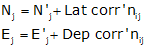### Article Index

The traverse misclosure is determined by comparing the preliminary coordinates of the end point to its known starting coordinates:

Loop traverse, Figure I-1:Figure I-1 Loop Traverse Closure

On a loop traverse, the coordinates of the first point can be known or assumed.

The coordinates of the traverse endpoints must be known in the same system.

In this case E and H are known.Figure I-2 Link Traverse Closure

When the traverse is adjusted, corrections are applied directly to the preliminary coordinates (J') to obtain final coordinates (J), Figure I-3 and Equations E-3 and E-4.Figure I-3 Applying CorrectionsEquations E-3 and E-4

The line correction affects the line's endpoint, that is, the position of J changes relative to the position of I by the latitude and departure corrections of the line IJ.

Because the coordinates of J change, the coordinates of the next point, K, must change by the same amount plus the correction of the line JK, Figure I-4:Figure I-4 Cumulative Corrections

The position shift from K' to K'' is the same as the shifts from J' to J.This pattern of accumulating corrections continues until the final point where the adjusted coordinates should equal the known values.

Using the Compass Rule:Equations E-1 and E-2

To compute coordinate corrections, the equations are modified to:Equations I-1 and I-2

where ΔNi-1 and ΔEi-1 in each are the cumulative previous corrections.